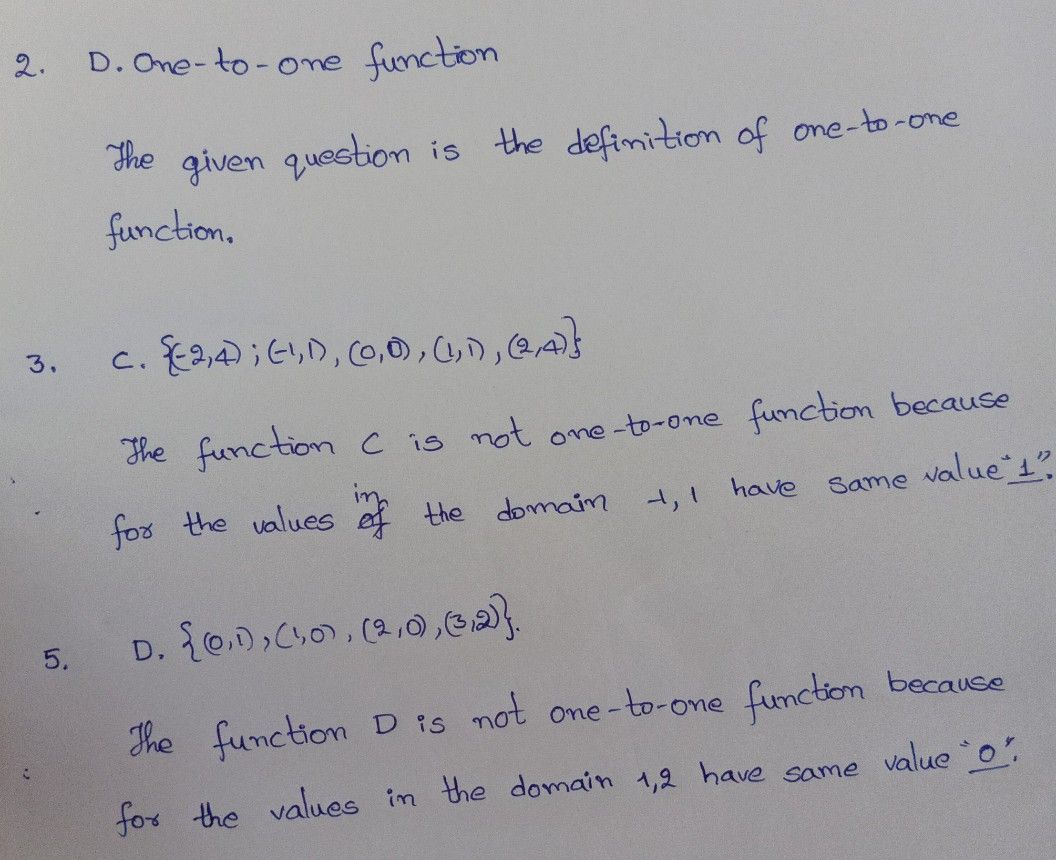Symbol
Problem$DirCctions3$ Write the letter of the correct answer on the space provided. If no answer is given, write the correct answer. of the function $P=\left(\left(-2,-3\right),\left(-2,-1\right),\left(-4,-1\right),\left(5,0\right)\right)$ $\bar{A.F^{1}\left(x} \right)=\left(\left(2.3\right).\left(2.1\right),\left(4.1\right),\left(-5.0\right)\right)$ $C.F^{1}\left(x\right)=\left(\left(3,2\right),\left(1,2\right),\left(1,4\right),\left(0,5\right)\right)$ $B.F^{1}\left(x\right)=\left(\left(-3,-2\right)\left(-1,-2\right).\left(-1,-4\right)\left(0,5\right)\right)$ $D.F^{1}\left(x\right)=\left(\left(-3.2\right),\left(1.2\right)\left(1,4\right),\left(0,5\right)\right)$ 2. It is a function in which for each value of y in the range of f, there is just one value x in the domain of f such that y = f(x). A. Constant Function C. Linear Function B. Identity Function D. $Onc-to-on$ Function function? 3. Which of the following is NOT a one-to-one $A.\left(\left(0,0\right),$ $\left(1,1\right),\left(2,8\right),\left(3,27\right),$ (4, 64)} $B.\left(\left(-2,6\right),$ (-1, 3), (0, 2), (1, 5), (2, 8)} $c.\left(\left(-2,4\right),$ (-1, 1), (0, 0), (1, 1), (2, 4)} D. $\left(0,4\right),\left(1,5\right),\left(2,6\right),\left(3,7...\left(n,n+4\right)...\right)\right)$ $\bar{A.0} ^{4.A}onc-to-on$ function crosses a horizontal line how many times? B. 1 C. 2 D. 3 5. Which of the following function is not $20c$ $10-02c$ A. {(0, 1), $\left(1,2\right),\left(2,3\right),\left(3,4\right)\right)$ C. {(0, $1\right),\left(1,0\right),\left(2,3\right)$ (3, 2)} B. $\left(\left(0,0\right),\left(1,1\right),\left(2,2\right),\left(3,3\right)\right)$ D. $\left(\left(0,1\right),\left(1,0\right),\left(2,0\right),\left(3,2\right)\right)$
10th-13th grade
Other
Search count: 135
Question content
Please help me answer only number 2,3 and 5 please thank you!
SolutionQanda teacher - D V MAHESHStudent
Number 2 is not an inverse function?Qanda teacher - D V MAHESH
yes an Inverse Function, but we have to go with the options. Also remember that every inverse function is a One-to-one function.Student
But in the direction it says "if there's no answer given, write the correct answer"Qanda teacher - D V MAHESH
then you can go with Inverse function.